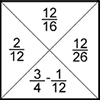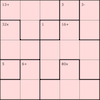# Number Operations & Calculation Methods### Consecutive Numbers

##### Age 7 to 14Challenge Level

An investigation involving adding and subtracting sets of consecutive numbers. Lots to find out, lots to explore.### Can You Make 100?

##### Age 11 to 14Challenge Level

How many ways can you find to put in operation signs (+, −, ×, ÷) to make 100?### Where Can We Visit?

##### Age 11 to 14Challenge Level

Charlie and Abi put a counter on 42. They wondered if they could visit all the other numbers on their 1-100 board, moving the counter using just these two operations: x2 and -5. What do you think?### Two and Two

##### Age 11 to 16Challenge Level

How many solutions can you find to this sum? Each of the different letters stands for a different number.### Number Daisy

##### Age 11 to 14Challenge Level

Can you find six numbers to go in the Daisy from which you can make all the numbers from 1 to a number bigger than 25?### The Root of the Problem

##### Age 14 to 18Challenge Level

Find the sum of this series of surds.### Remainders

##### Age 7 to 14Challenge Level

I'm thinking of a number. My number is both a multiple of 5 and a multiple of 6. What could my number be?### Largest Product

##### Age 11 to 14Challenge Level

Which set of numbers that add to 10 have the largest product?### Cinema Problem

##### Age 11 to 14Challenge Level

A cinema has 100 seats. Show how it is possible to sell exactly 100 tickets and take exactly Â£100 if the prices are Â£10 for adults, 50p for pensioners and 10p for children.### Consecutive Seven

##### Age 11 to 14Challenge Level

Can you arrange these numbers into 7 subsets, each of three numbers, so that when the numbers in each are added together, they make seven consecutive numbers?##### Age 7 to 14Challenge Level

Watch our videos of multiplication methods that you may not have met before. Can you make sense of them?### The Remainders Game

##### Age 7 to 14Challenge Level

Play this game and see if you can figure out the computer's chosen number.### Countdown

##### Age 7 to 14Challenge Level

Here is a chance to play a version of the classic Countdown Game.### Going Round in Circles

##### Age 11 to 14Challenge Level

Mathematicians are always looking for efficient methods for solving problems. How efficient can you be?### Missing Multipliers

##### Age 7 to 14Challenge Level

What is the smallest number of answers you need to reveal in order to work out the missing headers?### Cryptarithms

##### Age 11 to 14Challenge Level

Can you crack these cryptarithms?### Impossibilities

##### Age 11 to 14Challenge Level

Just because a problem is impossible doesn't mean it's difficult...### Overlaps

##### Age 11 to 14Challenge Level

Can you find ways to put numbers in the overlaps so the rings have equal totals?### Have You Got It?

##### Age 11 to 14Challenge Level

Can you explain the strategy for winning this game with any target?### Egyptian Fractions

##### Age 11 to 14Challenge Level

The Egyptians expressed all fractions as the sum of different unit fractions. Here is a chance to explore how they could have written different fractions.### Fractions Jigsaw

##### Age 11 to 14Challenge Level

A jigsaw where pieces only go together if the fractions are equivalent.### Round and Round and Round

##### Age 11 to 14Challenge Level

Where will the point stop after it has turned through 30 000 degrees? I took out my calculator and typed 30 000 ÷ 360. How did this help?### Twisting and Turning

##### Age 11 to 14Challenge Level

Take a look at the video and try to find a sequence of moves that will untangle the ropes.### More Twisting and Turning

##### Age 11 to 16Challenge Level

It would be nice to have a strategy for disentangling any tangled ropes...### Connect Three

##### Age 11 to 16Challenge Level

In this game the winner is the first to complete a row of three. Are some squares easier to land on than others?### Consecutive Negative Numbers

##### Age 11 to 14Challenge Level

Do you notice anything about the solutions when you add and/or subtract consecutive negative numbers?### Adding and Subtracting Positive and Negative Numbers

##### Age 11 to 14

How can we help students make sense of addition and subtraction of negative numbers?### Weights

##### Age 11 to 14Challenge Level

Different combinations of the weights available allow you to make different totals. Which totals can you make?### Keep it Simple

##### Age 11 to 14Challenge Level

Can all unit fractions be written as the sum of two unit fractions?### The Greedy Algorithm

##### Age 11 to 14Challenge Level

The Egyptians expressed all fractions as the sum of different unit fractions. The Greedy Algorithm might provide us with an efficient way of doing this.### Countdown Fractions

##### Age 11 to 16Challenge Level

Here is a chance to play a fractions version of the classic Countdown Game.### Magic Letters

##### Age 11 to 14Challenge Level

Charlie has made a Magic V. Can you use his example to make some more? And how about Magic Ls, Ns and Ws?### Strange Bank Account

##### Age 11 to 14Challenge Level

Imagine a very strange bank account where you are only allowed to do two things...### Up, Down, Flying Around

##### Age 11 to 14Challenge Level

Play this game to learn about adding and subtracting positive and negative numbers### Making a Difference

##### Age 11 to 14Challenge Level

How many different differences can you make?##### Age 11 to 14Challenge Level

Aisha's division and subtraction calculations both gave the same answer! Can you find some more examples?### Almost One

##### Age 11 to 14Challenge Level

Choose some fractions and add them together. Can you get close to 1?### 5 by 5 Mathdokus

##### Age 7 to 16Challenge Level

Can you use the clues to complete these 5 by 5 Mathematical Sudokus?